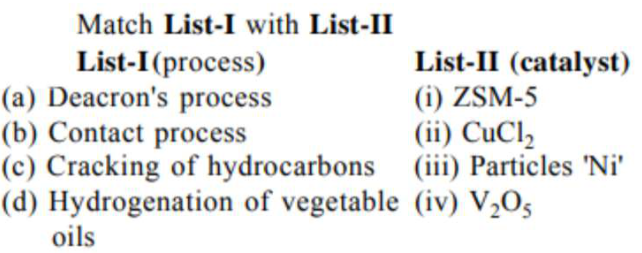# Match List-I with List-II

Question:Choose the most appropriate answer from the options given below -

1.  $a-i i, b-i v, c-i, d-i i i$

2. $\mathrm{a}-\mathrm{i}, \mathrm{b}-\mathrm{iii}, \mathrm{c}-\mathrm{ii}, \mathrm{d}-\mathrm{iv}$

3. $a-i i i, b-i, c-i v, d-i i$

4. $\mathrm{a}-\mathrm{iv}, \mathrm{b}-\mathrm{ii}, \mathrm{c}-\mathrm{i}, \mathrm{d}-\mathrm{iii}$

Correct Option: 1

Solution:

In manufacture of $\mathrm{H}_{2} \mathrm{SO}_{4}$ (contact process), $\mathrm{V}_{2} \mathrm{O}_{5}$ is used as a catalyst. $\mathrm{Ni}$ catalysts enables the hydrogenation of fats. $\mathrm{CuCl}_{2}$ is used as catalyst in Deacon's process. ZSM-5 used as catalyst in cracking of hydrocarbons.